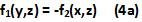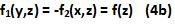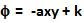## Saturday, July 3, 2010

### Irodov Problem 3.48

We know that,From the problem definition we have,Integrating both sides of each of the set of simultaneous equations (2a), (2b) (2c) we have,Now we have to find f1(y,z), f2(x,z) and f3(x,y) such that all the equations (3a),(3b) and (3c) are simultaneously satisfied.

Subtracting (3a) and (3b) we get,Equation (4a) can only be true ifIn other words f1 and f2 must be only functions of z and must be equal and opposite. Hence, now we have,Now subtracting (3c) and (5) we obtain,Now (6) can only be true if and only if,Hence, we have,